## An inflatable balloon with the volume of 0.75 L at 303 K was placed inside the freezer where the temperature is 263 K. Find out what will ha

Question

An inflatable balloon with the volume of 0.75 L at 303 K was placed inside the freezer where the temperature is 263 K. Find out what will happen to the volume of the balloon if the pressure remains constant.

in progress 0
6 months 2021-07-23T06:04:14+00:00 1 Answers 4 views 0

## Answers ( )

Final volume, V2 = 0.66 Litres

Explanation:

Given the following data;

Initial volume = 0.75 L

Initial temperature = 303 K

Final temperature = 263 K

To find out what will happen to the volume of the balloon if the pressure remains constant, we would use Charles’ law;

Charles states that when the pressure of an ideal gas is kept constant, the volume of the gas is directly proportional to the absolute temperature of the gas.

Mathematically, Charles’ law is given by the formula;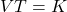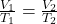Where;

• V1 and V2 represents the initial and final volumes respectively.
• T1 and T2 represents the initial and final temperatures respectively.

Making V2 as the subject formula, we have;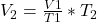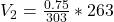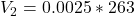Final volume, V2 = 0.6575 ≈ 0.66 Litres